# 8 1 Identifying Quadratic Functions Warm Up Lesson

• Slides: 34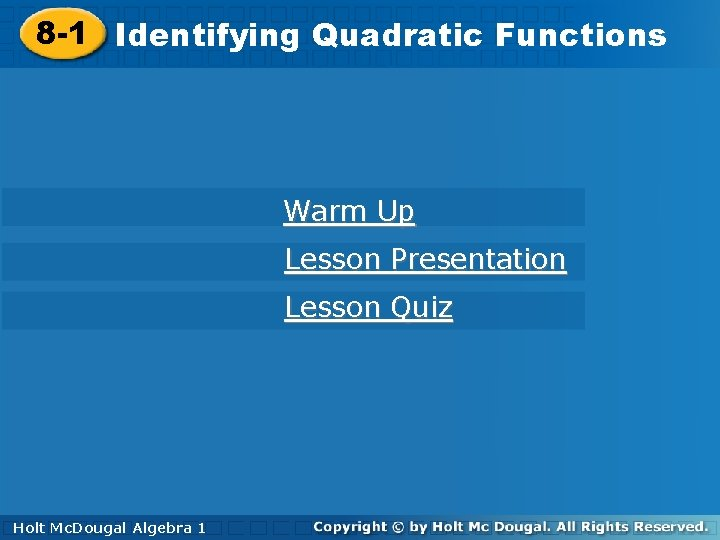8 -1 Identifying. Quadratic. Functions Warm Up Lesson Presentation Lesson Quiz Holt Mc. Dougal Algebra 1 Algebra 11 Holt Mc. Dougal8 -1 Identifying Quadratic Functions Warm Up 1. Evaluate x 2 + 5 x for x = 4 and x = – 3. 36; – 6 2. Generate ordered pairs for the function y = x 2 + 2 with the given domain. D: {– 2, – 1, 0, 1, 2} x – 2 – 1 0 1 2 y 6 3 2 3 6 Holt Mc. Dougal Algebra 1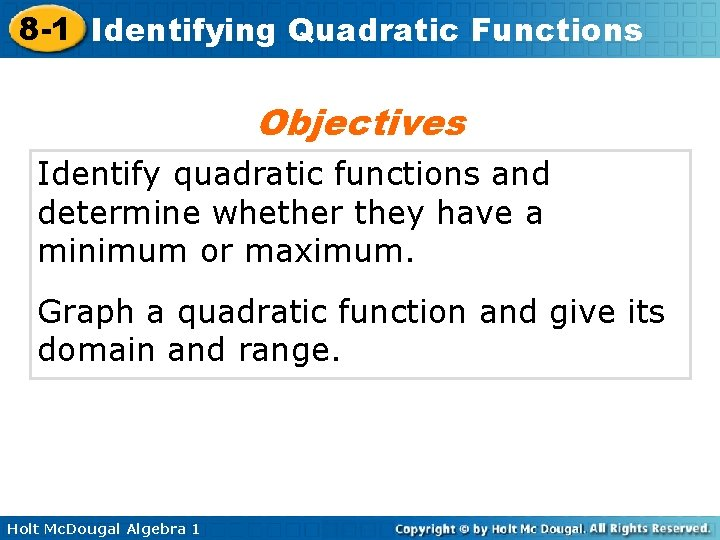8 -1 Identifying Quadratic Functions Objectives Identify quadratic functions and determine whether they have a minimum or maximum. Graph a quadratic function and give its domain and range. Holt Mc. Dougal Algebra 1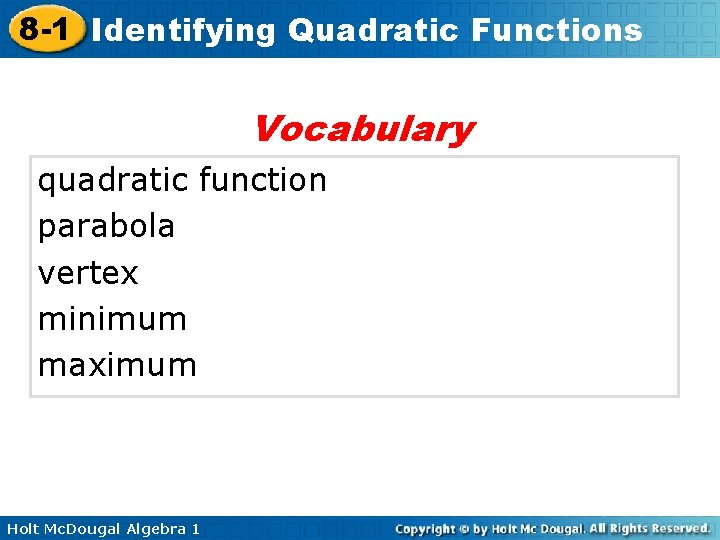8 -1 Identifying Quadratic Functions Vocabulary quadratic function parabola vertex minimum maximum Holt Mc. Dougal Algebra 18 -1 Identifying Quadratic Functions The function y = x 2 is shown in the graph. Notice that the graph is not linear. This function is a quadratic function. A quadratic function is any function that can be written in the standard form y = ax 2 + bx + c, where a, b, and c are real numbers and a ≠ 0. The function y = x 2 can be written as y = 1 x 2 + 0 x + 0, where a = 1, b = 0, and c = 0. Holt Mc. Dougal Algebra 18 -1 Identifying Quadratic Functions In Lesson 5 -1, you identified linear functions by finding that a constant change in x corresponded to a constant change in y. The differences between yvalues for a constant change in x-values are called first differences. Holt Mc. Dougal Algebra 18 -1 Identifying Quadratic Functions Notice that the quadratic function y = x 2 doe not have constant first differences. It has constant second differences. This is true for all quadratic functions. Holt Mc. Dougal Algebra 1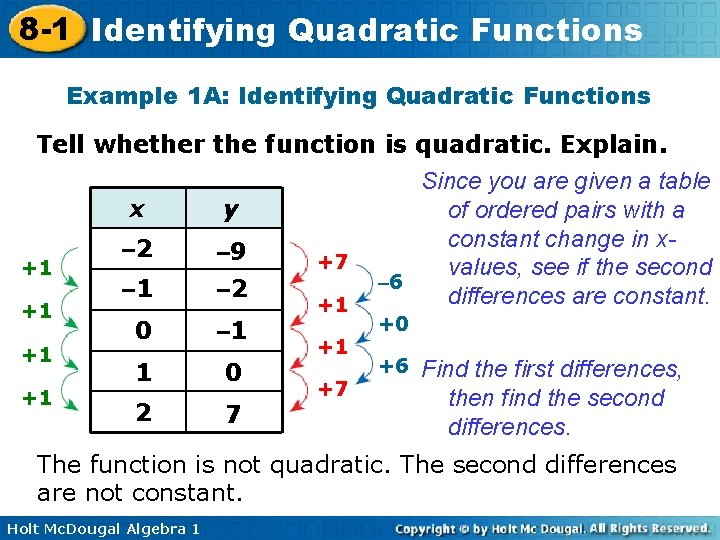8 -1 Identifying Quadratic Functions Example 1 A: Identifying Quadratic Functions Tell whether the function is quadratic. Explain. Since you are given a table x y of ordered pairs with a constant change in x– 2 – 9 +7 +1 values, see if the second – 6 – 1 – 2 differences are constant. +1 +1 +0 – 1 0 +1 +1 1 0 2 7 +1 +7 +6 Find the first differences, then find the second differences. The function is not quadratic. The second differences are not constant. Holt Mc. Dougal Algebra 1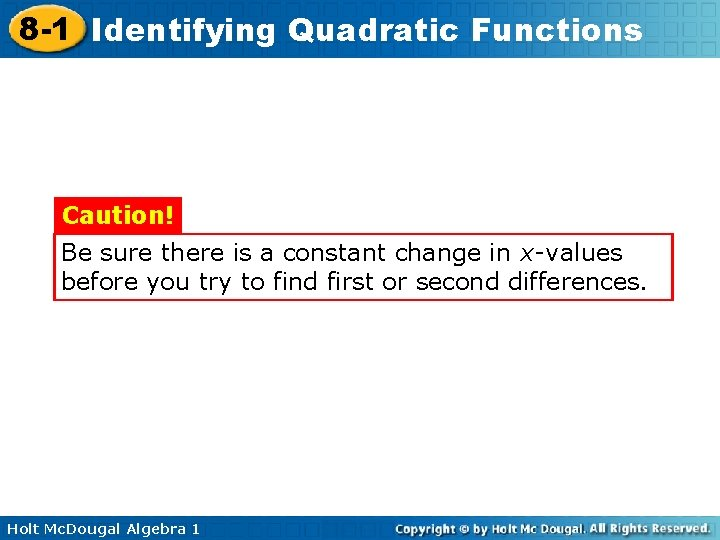8 -1 Identifying Quadratic Functions Caution! Be sure there is a constant change in x-values before you try to find first or second differences. Holt Mc. Dougal Algebra 1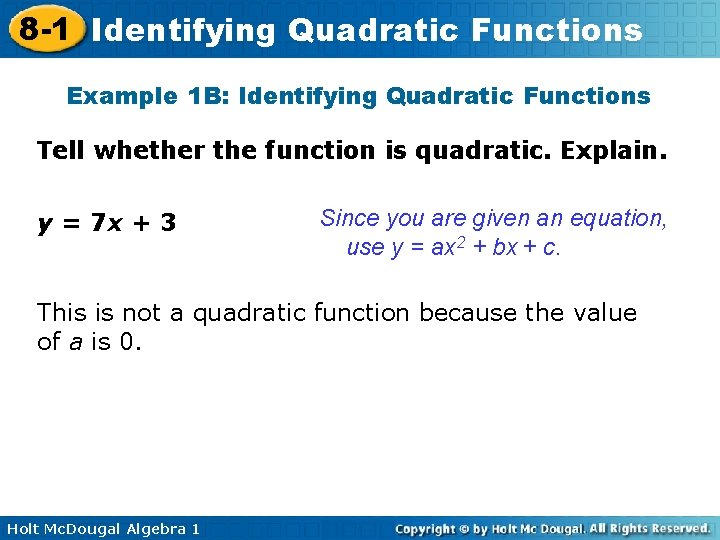8 -1 Identifying Quadratic Functions Example 1 B: Identifying Quadratic Functions Tell whether the function is quadratic. Explain. y = 7 x + 3 Since you are given an equation, use y = ax 2 + bx + c. This is not a quadratic function because the value of a is 0. Holt Mc. Dougal Algebra 1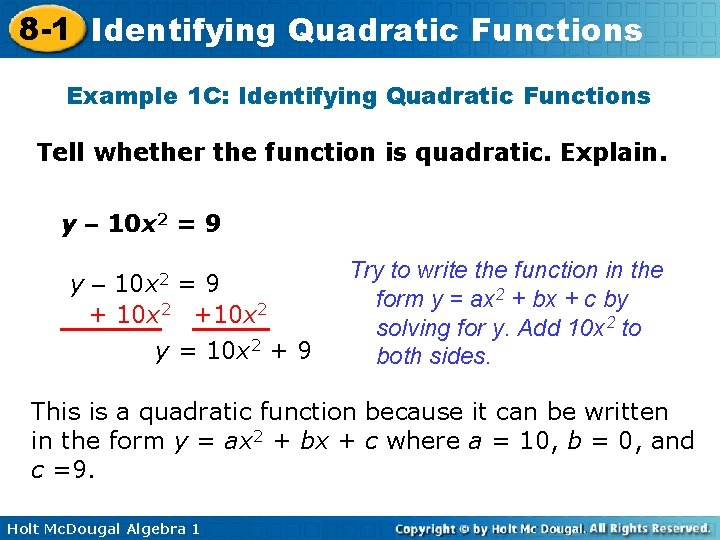8 -1 Identifying Quadratic Functions Example 1 C: Identifying Quadratic Functions Tell whether the function is quadratic. Explain. y – 10 x 2 = 9 10 x 2 y– =9 + 10 x 2 +10 x 2 y = 10 x 2 + 9 Try to write the function in the form y = ax 2 + bx + c by solving for y. Add 10 x 2 to both sides. This is a quadratic function because it can be written in the form y = ax 2 + bx + c where a = 10, b = 0, and c =9. Holt Mc. Dougal Algebra 18 -1 Identifying Quadratic Functions Helpful Hint In a quadratic function, only a cannot equal 0. It is okay for the values of b and c to be 0. Holt Mc. Dougal Algebra 18 -1 Identifying Quadratic Functions Check It Out! Example 1 a Tell whether the function is quadratic. Explain. {(– 2, 4), (– 1, 1), (0, 0), (1, 1), (2, 4)} List the ordered pairs in a x y table of values. Since there is a constant – 2 4 – 3 +1 change in the x-values, +2 – 1 1 see if the differences are – 1 +1 constant. +2 0 0 +1 +1 1 1 2 4 +1 +3 +2 Find the first differences, then find the second differences. The function is quadratic. The second differences are constant. Holt Mc. Dougal Algebra 18 -1 Identifying Quadratic Functions Check It Out! Example 1 b Tell whether the function is quadratic. Explain. y + x = 2 x 2 –x –x y = 2 x 2 – x Try to write the function in the form y = ax 2 + bx + c by solving for y. Subtract x from both sides. This is a quadratic function because it can be written in the form y = ax 2 + bx + c where a = 2, b = – 1, and c = 0. Holt Mc. Dougal Algebra 18 -1 Identifying Quadratic Functions The graph of a quadratic function is a curve called a parabola. To graph a quadratic function, generate enough ordered pairs to see the shape of the parabola. Then connect the points with a smooth curve. Holt Mc. Dougal Algebra 18 -1 Identifying Quadratic Functions Example 2 A: Graphing Quadratic Functions by Using a Table of Values Use a table of values to graph the quadratic function. x y – 2 4 3 1 3 – 1 0 1 2 0 1 3 4 3 Holt Mc. Dougal Algebra 1 Make a table of values. Choose values of x and use them to find values of y. Graph the points. Then connect the points with a smooth curve.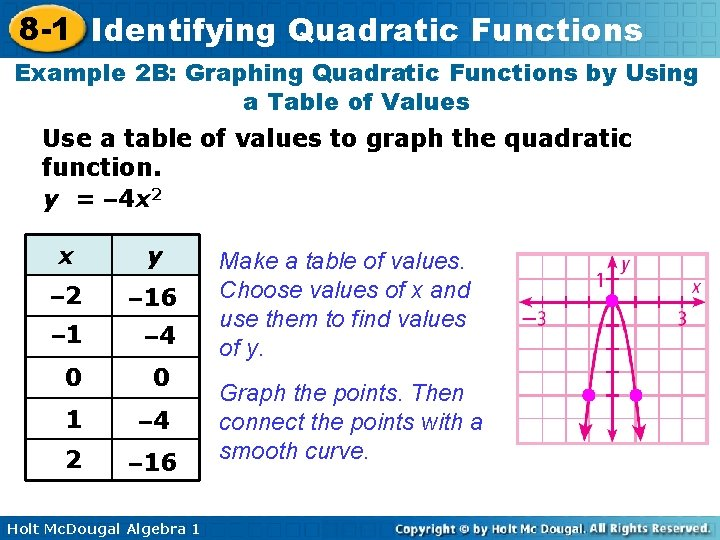8 -1 Identifying Quadratic Functions Example 2 B: Graphing Quadratic Functions by Using a Table of Values Use a table of values to graph the quadratic function. y = – 4 x 2 x y – 2 – 16 – 1 – 4 0 0 1 – 4 2 – 16 Holt Mc. Dougal Algebra 1 Make a table of values. Choose values of x and use them to find values of y. Graph the points. Then connect the points with a smooth curve.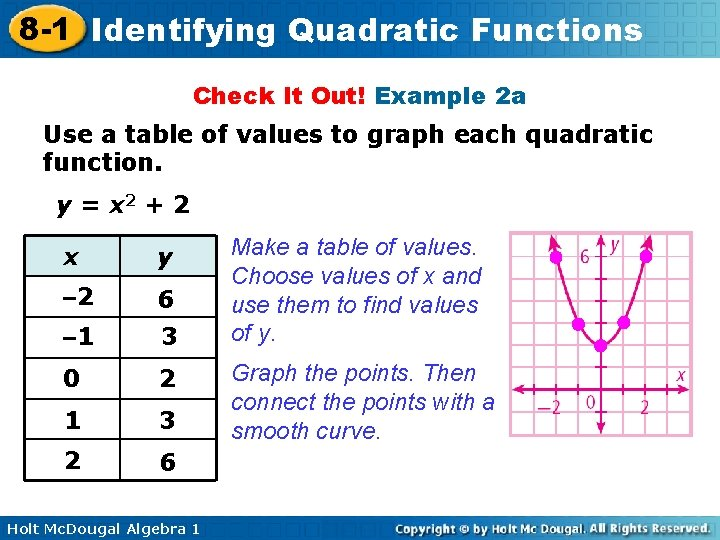8 -1 Identifying Quadratic Functions Check It Out! Example 2 a Use a table of values to graph each quadratic function. y = x 2 + 2 x y – 2 6 – 1 3 0 2 1 3 2 6 Holt Mc. Dougal Algebra 1 Make a table of values. Choose values of x and use them to find values of y. Graph the points. Then connect the points with a smooth curve.8 -1 Identifying Quadratic Functions Check It Out! Example 2 b Use a table of values to graph the quadratic function. y = – 3 x 2 + 1 x y – 2 – 11 – 2 0 1 1 – 2 2 – 11 Holt Mc. Dougal Algebra 1 Make a table of values. Choose values of x and use them to find values of y. Graph the points. Then connect the points with a smooth curve.8 -1 Identifying Quadratic Functions As shown in the graphs in Examples 2 A and 2 B, some parabolas open upward and some open downward. Notice that the only difference between the two equations is the value of a. When a quadratic function is written in the form y = ax 2 + bx + c, the value of a determines the direction a parabola opens. • A parabola opens upward when a > 0. • A parabola opens downward when a < 0. Holt Mc. Dougal Algebra 1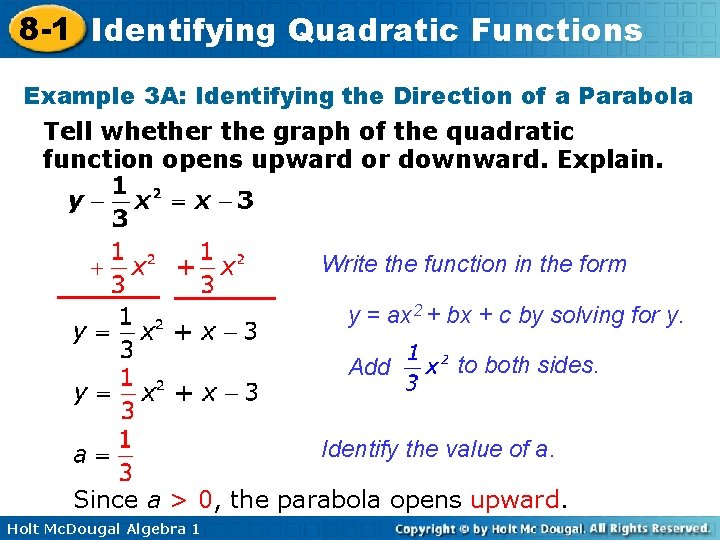8 -1 Identifying Quadratic Functions Example 3 A: Identifying the Direction of a Parabola Tell whether the graph of the quadratic function opens upward or downward. Explain. Write the function in the form y = ax 2 + bx + c by solving for y. Add to both sides. Identify the value of a. Since a > 0, the parabola opens upward. Holt Mc. Dougal Algebra 1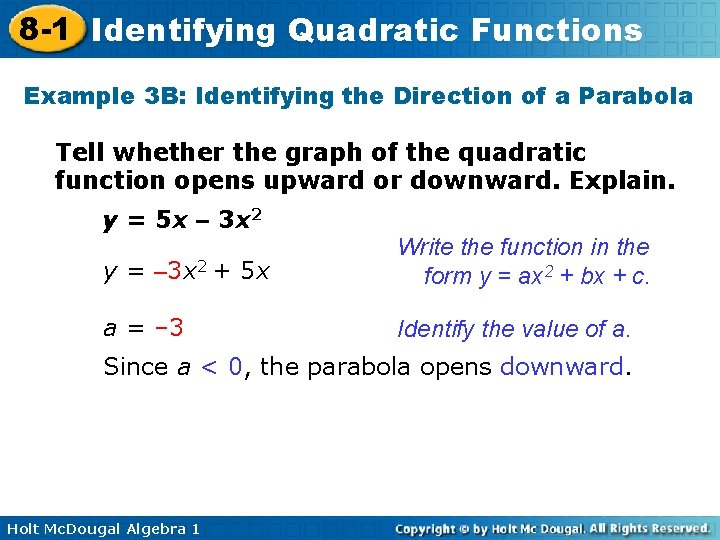8 -1 Identifying Quadratic Functions Example 3 B: Identifying the Direction of a Parabola Tell whether the graph of the quadratic function opens upward or downward. Explain. y = 5 x – 3 x 2 y = – 3 x 2 + 5 x Write the function in the form y = ax 2 + bx + c. a = – 3 Identify the value of a. Since a < 0, the parabola opens downward. Holt Mc. Dougal Algebra 18 -1 Identifying Quadratic Functions Check It Out! Example 3 a Tell whether the graph of the quadratic function opens upward or downward. Explain. f(x) = – 4 x 2 – x + 1 a = – 4 Identify the value of a. Since a < 0 the parabola opens downward. Holt Mc. Dougal Algebra 1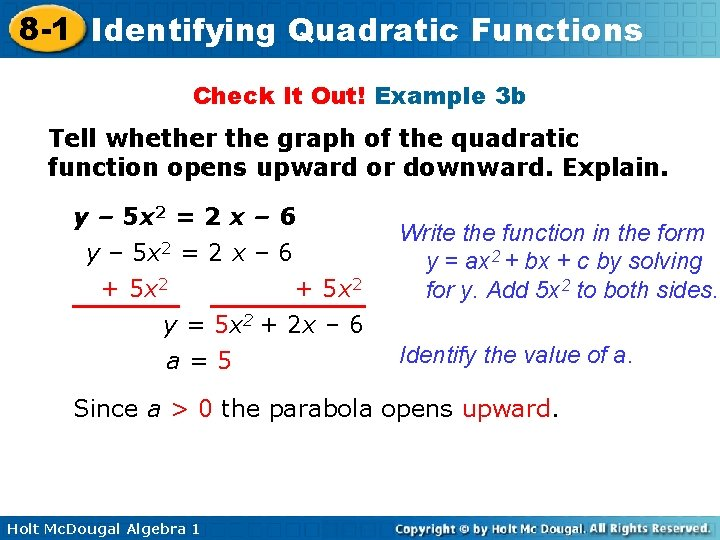8 -1 Identifying Quadratic Functions Check It Out! Example 3 b Tell whether the graph of the quadratic function opens upward or downward. Explain. y – 5 x 2 = 2 x – 6 + 5 x 2 y = 5 x 2 + 2 x – 6 a=5 Write the function in the form y = ax 2 + bx + c by solving for y. Add 5 x 2 to both sides. Identify the value of a. Since a > 0 the parabola opens upward. Holt Mc. Dougal Algebra 1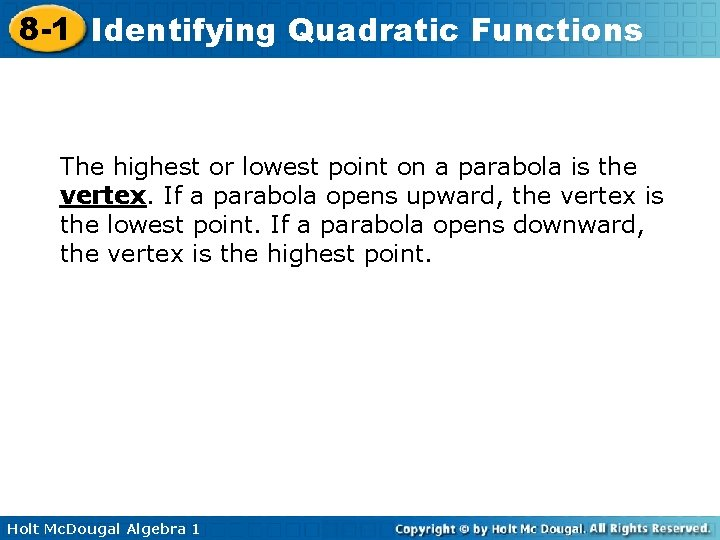8 -1 Identifying Quadratic Functions The highest or lowest point on a parabola is the vertex. If a parabola opens upward, the vertex is the lowest point. If a parabola opens downward, the vertex is the highest point. Holt Mc. Dougal Algebra 1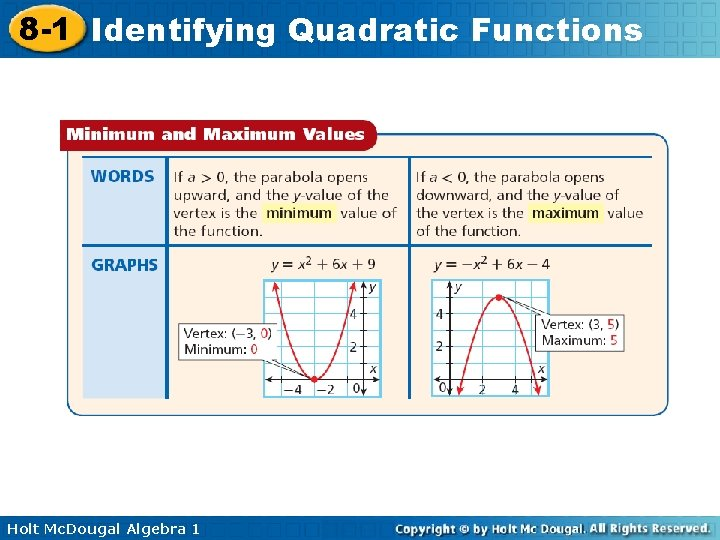8 -1 Identifying Quadratic Functions Holt Mc. Dougal Algebra 18 -1 Identifying Quadratic Functions Example 4: Identifying the Vertex and the Minimum or Maximum Identify the vertex of each parabola. Then give the minimum or maximum value of the function. A. B. The vertex is (– 3, 2), and the minimum is 2. Holt Mc. Dougal Algebra 1 The vertex is (2, 5), and the maximum is 5.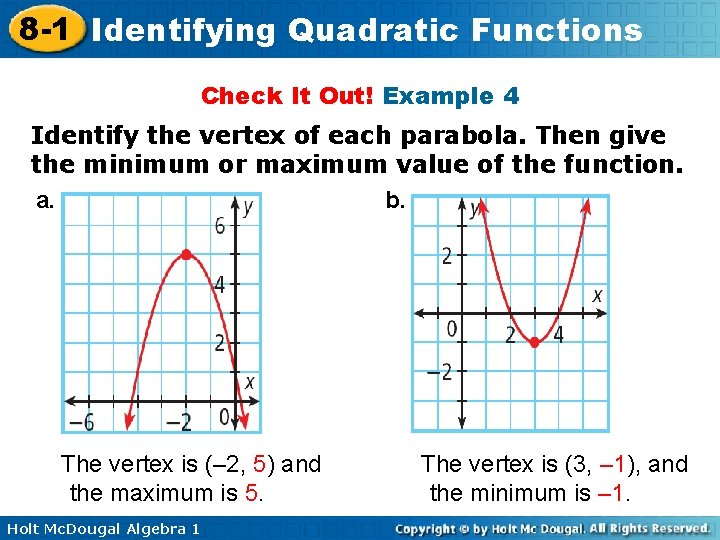8 -1 Identifying Quadratic Functions Check It Out! Example 4 Identify the vertex of each parabola. Then give the minimum or maximum value of the function. a. b. The vertex is (– 2, 5) and the maximum is 5. Holt Mc. Dougal Algebra 1 The vertex is (3, – 1), and the minimum is – 1.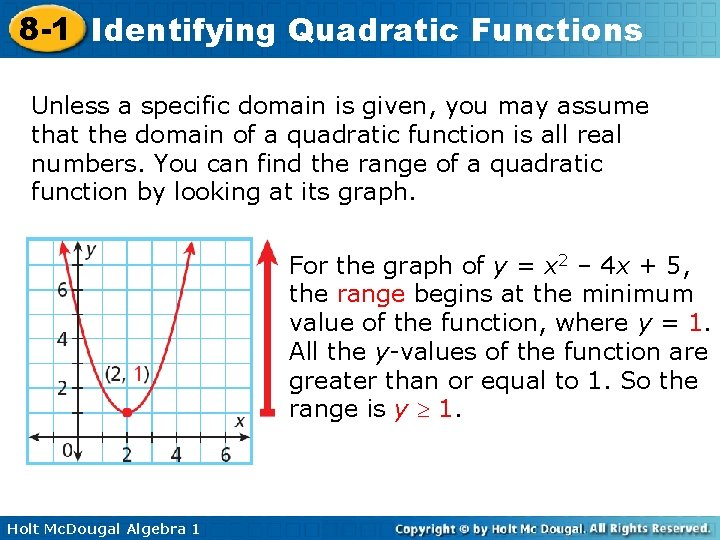8 -1 Identifying Quadratic Functions Unless a specific domain is given, you may assume that the domain of a quadratic function is all real numbers. You can find the range of a quadratic function by looking at its graph. For the graph of y = x 2 – 4 x + 5, the range begins at the minimum value of the function, where y = 1. All the y-values of the function are greater than or equal to 1. So the range is y 1. Holt Mc. Dougal Algebra 18 -1 Identifying Quadratic Functions Example 5: Finding Domain and Range Find the domain and range. Step 1 The graph opens downward, so identify the maximum. The vertex is (– 5, – 3), so the maximum is – 3. Step 2 Find the domain and range. D: all real numbers R: y ≤ – 3 Holt Mc. Dougal Algebra 18 -1 Identifying Quadratic Functions Check It Out! Example 5 a Find the domain and range. Step 1 The graph opens upward, so identify the minimum. The vertex is (– 2, – 4), so the minimum is – 4. Step 2 Find the domain and range. D: all real numbers R: y ≥ – 4 Holt Mc. Dougal Algebra 18 -1 Identifying Quadratic Functions Check It Out! Example 5 b Find the domain and range. Step 1 The graph opens downward, so identify the maximum. The vertex is (2, 3), so the maximum is 3. Step 2 Find the domain and range. D: all real numbers R: y ≤ 3 Holt Mc. Dougal Algebra 1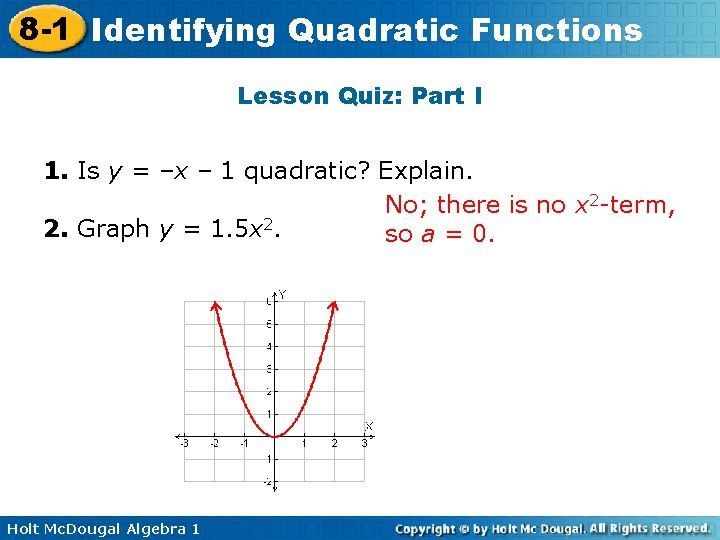8 -1 Identifying Quadratic Functions Lesson Quiz: Part I 1. Is y = –x – 1 quadratic? Explain. No; there is no x 2 -term, 2. Graph y = 1. 5 x 2. so a = 0. Holt Mc. Dougal Algebra 18 -1 Identifying Quadratic Functions Lesson Quiz: Part II Use the graph for Problems 3 -5. 3. Identify the vertex. (5, – 4) 4. Does the function have a minimum or maximum? What is it? max; – 4 5. Find the domain and range. D: all real numbers; R: y ≤ – 4 Holt Mc. Dougal Algebra 1